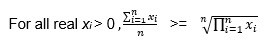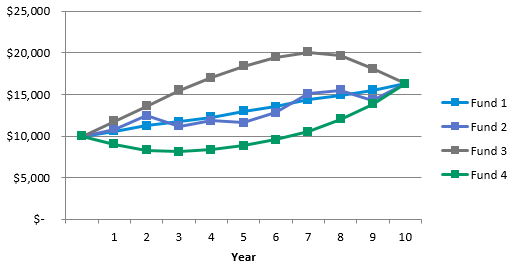Practical analysis for investment professionals
25 July 2018# The Myth of Volatility Drag (Part 2)

For a post on volatility drag, I sure didn’t spend much time talking about it in the first installment. I’ll rectify that now.

The myth of volatility drag takes two forms, Simple and Sophisticated. Simple is appealing because it matches our instincts and seems so clear that it must be true. Sophisticated looks daunting.

### Define the Relationship

In Thinking, Fast and Slow, Daniel Kahneman describes “fast,” or System 1, thinking as largely unconscious and the way we first try to make sense of the world. It lets us operate quickly with incomplete information.

Our “slow,” System 2, thinking, on the other hand, requires much more effort and we only apply it for close analysis and to reflect on assumptions. In this framing, the expectations we form during “fast” thinking can trip up our “slow” analysis if we don’t sufficiently examine them.

Holding the arithmetic mean of annual returns equal, a security with lower volatility will have higher compound growth.

True.

The difference between them is volatility drag.

What? Calling it “drag” creates an association since “drag” is generally understood as a force. But there is no “force” here, merely a mathematical relationship. Volatility’s negative connotations are already there: We’ve been primed to equate volatility with “risk.” Indeed, a related question discussed in Enterprising Investor is whether volatility is really a good measure of risk.

Contrast that with this statement:

The difference between them is volatility inflation, because the volatility “inflates” the arithmetic mean.

“Inflation” is no less accurate than “drag,” it’s simply a new name with new associations. Neither set of associations is particularly helpful because the real difference lies in the relationship of the functions themselves. The following inequality equation defines the relationship of the arithmetic and geometric means.Consider an example with two values, a and b, where 10. The arithmetic average for all {a,b} is therefore 10/2 = 5.

It is less obvious what the geometric mean will be, so we’ll try a few values. If we take a = 3 and b = 7, 3*7 = 21, √21 ≈ 4.58.If we apply this to all a and b, we end up with a parametric definition of a half circle:

a+ b= r2, where r is the radius of a circle.

In the diagram below, the values a and b may vary along the x axis and the means, both geometric and arithmetic, are plotted on the y axis. The dashed lines show the particular example of a = 3 and b = 7.

Averaging “A” and “B”One might reply, “So what?” And that may be the point. It may not mean anything, it’s just the way the functions relate.

### We’re not talking about apples.

Simple goes like this:

• Invest \$100, the price goes down 10% on day 1, and up 10% on day 2, but we end up with 99%, or \$99.00, at the end of day 2. The average of 10% and -10% is 0%, but the total return was -1%, so volatility drag ate 1%.
• Sometimes a “proof” is offered: If = daily return, then (1 – x)(1 + x) = 1 – x2, for all x not equal to zero, we end up with less than we started, thus “proving” that volatility “causes” lost value.

Thinking fast, the pitfall is to treat “percentages” as if they were apples. “If we have 100 apples, then lose 10 apples, then gain 10 apples, how many apples do we have?” We know the answer is 100 apples because we’ve experienced many analogous situations. When we slow down, we recall that percentage is not an absolute but a relative measurement. The denominator is changing. Ten percent of 100 is 10, so \$110 after the first day. Ten percent of 110 is 11, so \$99 after the second day.

The algebraic form is the same, just better hidden. Multiplying (1 – x) and (1 + x) is executed correctly, but then assigns inaccurate meaning to this equation. As we reviewed in Part 1, this is a product so the arithmetic mean of the elements is not meaningful:

The geometric mean gives the actual daily return.√((1 – .1)(1 + .1)) ≈ .995 or a daily return of -0.5%.

Let’s put this back in securities terms by following four funds. On a 10-year horizon, they all end up at exactly the same place. Each fund has the same geometric mean, 5%. Can we measure their performance over the time period with the geometric or arithmetic mean?

Hypothetical Growth of Four FundsFunds 2, 3, and 4 all have the same arithmetic mean of returns. They also all each have the same variance, which is higher than Fund 1. But Fund 1 does not appear to have benefited or suffered by comparison. How is that possible? There are infinite paths between the start and end point. In this case, the annual returns for funds 2, 3, and 4 are all the same numbers, just arranged in different orders.### Sophisticated: The Standard Weiner Process

The Sophisticated version is more beguiling, but ultimately makes the same misstep as its Simple counterpart: Our fast system creates an expectations mismatch by applying a familiar idea and running it through a less a familiar mathematical construct. Consider the “Random Walk” pricing model where the standard Weiner process (sometimes called Brownian Motion) is our random variable.

Suppose that security prices can be modeled with the following differential equation, where P is the price, μ is the expected change in price of the stock over change in time dt, σ is the standard deviation of price, and z is a standard Weiner process.

dP = μP dt + σP dz

The salient feature our fast system trips on is here: The symbol μ is used, as is conventional, to represent the arithmetic mean of a data set or the expected value of a random variable. More importantly, is expected over change in time, dt. The important element to note is that it is not all time.

Now we can identify a mismatch of definition vs. expectation. In the pricing model, we input the arithmetic mean of the price changes over some time frame, say daily. This can create an expectation that the output will compound at that rate. After all, our savings accounts, CDs, and other fixed-return products do. Our slow system lets us recall that when we chain the prices, by calculating the prices on days 2, 3, etc., their relationship is a product, not a summation. Thus, the geometric mean is the correct “average” used to project the resulting growth rate over the full time period of the analysis.

### Strategies Based on Volatility Drag

To be very, very clear, any given product may be excellent and its return behaviors may match what an investor wants. There could be other reasons beyond seeking excess performance to want low-volatility portfolios. And when seeking excess performance, I do not argue that volatility is impossible to leverage in such investment strategies.

“Buy low, sell high” still applies. I want to convince you that performance is not caused by minimizing volatility itself. My stretch goal? Let’s banish “volatility drag” from our vocabularies!

If you liked this post, don’t forget to subscribe to the Enterprising Investor.

All posts are the opinion of the author. As such, they should not be construed as investment advice, nor do the opinions expressed necessarily reflect the views of CFA Institute or the author’s employer.

Share On
##### Will Morrison

Will Morrison is manager, business analytics and reporting, at CFA Institute. Previously, he was an engineer at GE, specializing in robotic path planning. Morrison has earned the Claritas Investment Certificate. He holds a BA in computer science from Goucher College, a BS in electrical engineering from the Johns Hopkins University, and a masters of systems engineering from the University of Virginia.

## 5 thoughts on “The Myth of Volatility Drag (Part 2)”

1.David Harper says:

Confusing (sorry). Volatility drag a real thing. If you start with \$100, at the end of N periods, your CAGR (aka, geometric mean with annual compounding) is approx σ^2/2 less the arithmetic mean. Your concern is the dollars you have at then end of the period, and CAGR reflects this, and incorporates the volatility drag.

1.Matt Crouse says:

I agree with the other commentator. For example, consider the following quote from the article: “I want to convince you that performance is not caused by minimizing volatility itself. ”

Although obviously volatility is not the only cause of performance, it is one of the determining factors. Booth and Fama in their article “Diversification Returns and Asset Contributions” (published in the FAJ!) demonstrate that you can improve the compounded returns of a portfolio by decreasing its volatility. This seems to directly contradict the author’s claim.

One can validly argue the semantics of what to call the difference between arithmetic returns and geometric returns, but we should understand the difference and its underlying causes. Given the appellation “volatility drag” is already in widespread use, I see no reason to discontinue its use, as the arguments against it seem more philosophical than practical.

2.Matthew Crouse says:

I am publishing an article on volatility drag ETFs in the Journal of Index Investing showing (among other things) that monthly leverage rebalancing in leveraged ETF improves returns over daily leverage rebalancing due to guess what — lower volatility and, therefore, lower volatility drag.

https://doi.org/10.3905/jii.2019.1.074

I originally submitted this to the CFA’s Financial Analyst Journal (FAJ), but the FAJ rejected the paper in part because of this unrefereed blog by Mr. Morrison that says volatility drag doesn’t even exist. Needless to say, that was very frustrating given the nonexistence claim is really just an argument of semantics. There is no debate over the underlying math/statistics.

2.Pro Quant Investor says:

Hey, Will,
Good effort to explain this and thanks for taking the effort… but the writing is confusing unless one is already familiar with the concept. Also, parts 1 and 2 taken together are meandering. Volatility drag is not a “myth”, unless you play with the semantics as you’re doing in this article. Calling it “drag” as opposed to “inflation” focuses on the effect on returns, which is what people care about. “Inflation” puts the focus on the volatility itself as both cause and effect. If the effect on returns is not implicit in the phrase, most people ask, “So what?”

If the goal is to teach someone, I think the better way is to show the lower CAGR of a high vol return series with an example early on, and then explain the math behind it without resorting to the Wiener process, which is unnecessary. Anybody who knows about stochastic vol wouldn’t need to read this, so why show it?

3.steve michael says:

love your article and it completely explains this so-called phenomenon…

two technical questions,

1) regarding a geometric mean, what about negative returns? i understand you can use (1+R/100), but it seems if the numbers are all positive, people use R instead.. will this theoretically give same result?………. can you use sqrt(R^n) somehow with negative numbers?

2) if i use norm.inv (norminv?) in excel, will that solve the problem? i’m thinking no as that’s normal, not lognormal